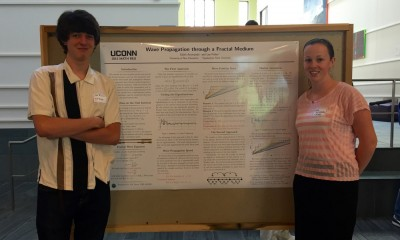# Group Members

Edith Aromando, Lee Fisher## Overview

We consider the wave equation on the unit interval with fractal measure, and use two numerical models to study wave speed and propagation distance.  The first approach uses a Fourier series of eigenfunctions of the fractal Laplacian, while the second uses a Markov chain to model the transmission and reflection of classical waves on an approximation of the fractal. These models have complementary advantages and limitations, and we conjecture that they approximate the same fractal wave.

Poster